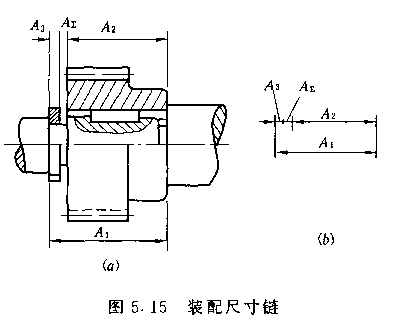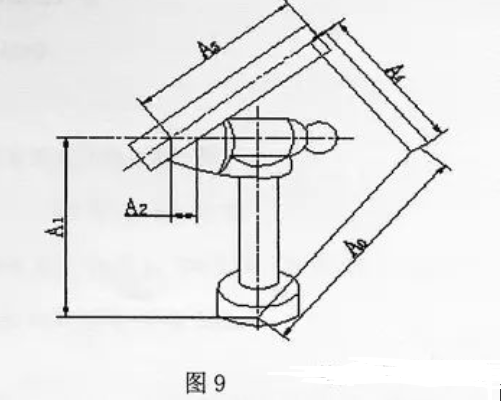## NEWS

### Company News

##### CONTACT MT

Contact person: Wendy  liu

Phone: (86) 15362889256

E-mail: ‍sales@dgmtwj.cn

Tel: (86) 769-88388276

wechat: +86 15362889256

Factory: No.2, Baidai Industrial Park, Daojiao Town, Dongguan, China ，

Hotline：(86) 15362889256

Current location： Homepage » NEWS » Industry News

# How to play with the size chain

Source: Browse：115 The release date：2022.04.20 [ Large medium small ]

Determination of Process Dimensions and Tolerances When Datum Coincidence

1. Determine the machining allowance for each machining operation

2. Starting from the last process, that is, from the design size to the first processing process, the allowance of each process is added one by one to obtain the basic dimensions of each process (including the blank size).

3. Except for the final process, the remaining processes determine the process dimensional tolerance according to the machining accuracy of the respective processing methods.

4. Mark the size of the process according to the principle of entering the body.（1） process dimension chain

Dimension chain definition:

Dimension chain: Connect the related dimensions in a certain order into a closed figure connected end to end.

Process dimension chain: A dimension chain of related dimensions formed by a single part in the process.

The composition of the size chain

1. Ring: Each size A1, A2, , A3 that make up the size chain

2. Closed ring: dimension A2 obtained indirectly during machining.

3. Forming ring: dimensions A1 and A3 obtained directly during processing.

Increased ring: The other constituent rings remain unchanged, and the increase of this ring increases the closed ring.

Subtraction ring: The other constituent rings remain unchanged, and the increase of this ring reduces the closed ring.

Specific judgment: choose a direction for the closed ring, turn it around in this direction, and add a direction to each ring. The same direction as the closed ring is the reduction ring, and the opposite is the increase ring.

Features:

1. The inch chain must be closed

2. Dimensional chains have only one closed loop

3. The accuracy of the closed loop is lower than the accuracy of the constituent loop

4. The closed ring changes with the composition of the ring

practice:

1. find closed loop

2. From the closed loop, draw the constituent loops in sequence according to the relationship on the surface of the workpiece, until the size returns to the closed loop to form a closed figure, and the number of constituent loops that make up the dimension chain should be the least.

3. The principle of connection is to determine the increase and decrease of the ring.

（2）Basic Calculation of Dimension Chains

1. Each dimension in the dimension chain diagram is called a link, and all the links constitute a dimension chain.

2. In the size chain, the size that can be controlled or obtained directly is called the composition ring.

3. The dimension obtained indirectly in the dimension chain, and the dimension formed naturally when other dimensions appear is called a closed ring (terminal ring). Each increase or decrease of the constituent loops changes the closed loop, and there is only one closed loop in a dimension chain.

4. The increase of a constituent ring while the other constituent rings remain unchanged will cause the closed ring to increase accordingly, then this constituent ring is an increasing ring. (The direction opposite to the closed loop is the increasing loop.)

5. The increase of a constituent ring while the other constituent rings remain unchanged will reduce the closed ring, then this constituent ring is a reduced ring. (The one that is in the same direction as the closed loop is the minus loop.)（3） Calculation formulas

The basic size of the closed ring = the algebraic sum of the basic size of each constituent ring - the sum of the basic size of the added ring - the sum of the basic size of the subtracted ring.

The maximum limit size of the closed ring = the maximum limit size of all added rings - the smallest limit size of all reduced rings.

Minimum limit size of closed ring = minimum limit size of all added rings - maximum limit size of all reduced rings.

Upper deviation of closed loop = sum of upper deviation of all added loops - sum of lower deviation of all subtracted loops

Lower deviation of closed loop = sum of lower deviation of all added loops - sum of upper deviation of all subtracted loops

Tolerance of closed ring = maximum limit size of closed ring - minimum limit size of closed ring

= upper deviation of the closed loop - lower deviation of the closed loop

= Sum of tolerances of increasing rings + sum of tolerances of decreasing rings

= Sum of tolerances of all constituent rings

Determination of deviation: generally based on the principle of 'into the body'

1) When the constituent ring is an inclusive surface, the lower deviation is 0, and the upper deviation = tolerance

2) When the constituent ring is a contained surface, the upper deviation is 0, and the lower deviation = tolerance